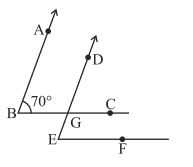Q

# In the given figure, the arms of two angles are parallel. If angle ABC = 70 degree then find angle DGC, angle DEF.

5. In the given figure, the arms of two angles are parallel. If , then findViews

(i) Since side AB is parallel to DG.

Thus :                           (Corresponding angles of parallel arms are equal.)

(ii)     Further side BC is parallel to EF.

We have :                             (Corresponding angles of parallel arms are equal.)

Exams
Articles
Questions